# Instruction Set of 8085 Microprocessor

An instruction is a binary command used to execute an operation inside the microprocessor over a given data. A group of instructions that are supported by the 8085 microprocessor is known to be the instruction set of 8085 microprocessor.

Basically, 8085 is designed to have 5 functional categories of the instruction set. Here in this article, we will discuss each instruction set in detail.

## Instruction set of 8085 microprocessor

We know that an instruction set is a group of instruction that executes the desired operation on the data specifically described by the microprocessor 8085.

The instruction set of 8085 microprocessor is classified as follows:Let us now move further to understand each instruction set separately.

### Data Transfer Instruction

These instructions are used by the microprocessor in order to transfer the data from one location to another. More specifically, it helps in transferring the data from source operand to destination operand, without making any changes in the source data.

As the data present in the source is not modified. Thus it is more convenient to call it a data copy instruction. As while transferring the data from source to destination, the data present in the source is just copied to the destination also. Due to this no change in source data is noticed.

Basically, the instructions that come under this instruction set, are as follows: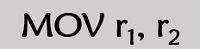This instruction helps to transfer the contents of register r2 into register r1. While the data in register r2 will remain the same.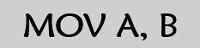This instruction transfers the data in register B to an accumulator.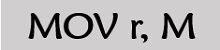This instruction specifies the transfer of data present in the memory to the register. However, the address of the memory location must be present in the HL register.

Example: MOV r, 1020HThis instruction specifies the immediate transfer of data to a memory location. And the address of this memory location is specified at the H-L registers.

Example: MOV M, 32H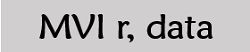In this instruction, the data is immediately transferred to the specified register.

Example: MVI r, 16HIt is load accumulator instruction. This instruction is used to copy the data present in the memory address specified as the operand of the instruction to the accumulator. More specifically, in this case, the data present in the 16-bit memory address is transferred to the accumulator.

Example: LDA 1000HIt is load accumulator indirect instruction. In this instruction, the register that acts as operand holds a memory location, where the actual data is present which is to be loaded at the accumulator.

Example: LDAX C/DIt stands for load H-L registers direct instruction. For this particular instruction, the data present in the address specified in the operand is copied to the L register. Also, the data present in the next memory location is loaded to the H register.

Example: LHLD 4500H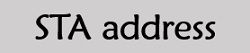STA is stored accumulator direct instruction. Whenever this instruction is passed, then the data present in the accumulator is transferred to the memory address specified in the operand.

Example: STA 2032HIt is stored accumulator indirect instruction. The register present as the operand holds a memory address. So, the data of the accumulator is copied to that particular memory location.

Example: STAX DThis instruction is used to exchange the data present in two registers.

Example: We have XCHG H-L and D-E

So the contents of H and D while L and E are exchanged.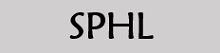This instruction transfers the data of HL pair into the stack pointer.Like the SPHL, this instruction copies the data of H-L register into the stack pointer by placing the higher order bytes at H and lower order bytes at L.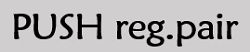In this instruction, the stack is loaded with the data present in the register given in the operand.

Firstly, the SP gets decremented and higher order bytes are copied to the stack. Further SP gets decremented in order to load the lower order register bytes.

Example: PUSH C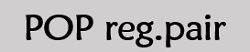This instruction specifies the transfer of the data present at the top of the stack to the register given as the operand.

Example: POP D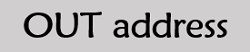In case of OUT instruction, the data at the accumulator is copied to the I/O port. At the operand an 8-bit port address is present.

Example: OUT 56 H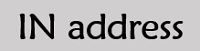This instruction specifies the loading of data present at the I/O port to the accumulator. The operand holds the address of the port from where the data is to be copied.

Example: IN, 5B H

### Arithmetic Instruction

This instruction set allows arithmetic operations to be performed over the data in memory and register inside the 8085 microprocessor.

The arithmetic instruction set is given as follows: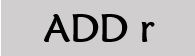This instruction specifies that the data present in the register is to be added with the data present in the accumulator and the result is to be stored in the accumulator itself.This instruction is used to add the data of the memory address specified at the operand with the data present at the accumulator. And the result of the addition is stored in the accumulator.In this particular instruction, the 8-bit data present as the operand is immediately added with the data at the accumulator and the result is stored at the accumulator.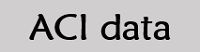This instruction adds the 8-bit data at the operand and carry flag with the data present at the accumulator. After each addition, the flag reflects the outcome of the addition.

Example: ACI 35H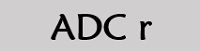In this instruction, the data at the register is added with the data present at the accumulator along with the carry bit and outcome is reflected at the accumulator.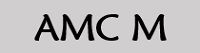This instruction is used to add the data present at the memory location whose address is specified in the operand given and the carry bit along with the data present in the accumulator. The result of the addition is stored in the accumulator.

Example: AMC 45H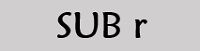This instruction subtracts the data present at the register given at the operand from the data in the accumulator, further the result is stored at the accumulator.

Example: SUB DIn this instruction, the data present at the memory location whose address is given by the H-L register is subtracted from the data at the accumulator.

Example: SUB 256HThis instruction is used to immediately subtract the data given as operand in the instruction from the data present at the accumulator. After each subtraction, the flag is modified so as to show the outcome of subtraction.

Example: SUI 55H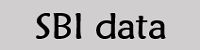This instruction subtracts the 8-bit data given as the operand and the borrow bit from the data present at the accumulator, and resultantly store the output achieved in the accumulator.

Example: SBI 21H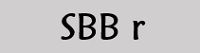By the use of this instruction, the data at the register and the borrow bit is subtracted from the data at the accumulator.

Example: SBB BThis instruction specifies the subtraction of data present at the memory location, whose address is present at the H-L register and the borrow bit from the data at the accumulator.

Example: SBB 1600H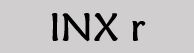This instruction increments the data by 1 which is present at the register given at the operand. The result of which is stored at the same register.

Example: INX D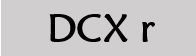This instruction decrements the data present at the register by 1 and stores the result at the same register.

Example: DCX DSometimes in an instruction, the operand holds a memory location. The address of this memory location is present at the H-L pair. So, the data present at that particular location is decremented by 1.

Example: DCR 42HIt stands for decimal adjust accumulator. This instruction causes to break the 8-bit binary number into two 4-bit binary coded decimal digits.

### Logical Instruction

These instructions are used to perform logical or Boolean operations over the data present in either register or memory. The logical instructions modify the flag bits according to the operation performed.

The logical instruction set is as follows: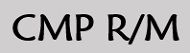This instruction is used to compare the data at the accumulator with the data present at the register or memory which is given as operand. According to the result obtained by the comparison, the flags are set. While the data being compared remains unchanged.

Suppose,

• If the data present at the accumulator is smaller than that of register or memory, then carry flag is set.
• If the data in the accumulator is equal to the data in the register or memory, then it sets the zero flag.
• However, if the data in the accumulator is greater than the data present in the memory or register, then both carry and zero flags are reset.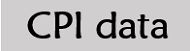This instruction is used to compare the 8-bit data given as operand in the instruction with the data present in the accumulator. The comparison result is shown by the flags.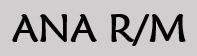This instruction performs the AND operation of the data present in the accumulator to the data present in the register or memory. After the AND operation, S, P, Z are changed to show the result of the comparison. AC is set and CY is reset.

Example: ANA  D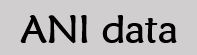This instruction is used to perform AND operation of the immediate 8-bit data given as operand with the data present in the accumulator.

Example: ANI 65HThis instruction executes OR operation, of the data in the accumulator with the data present in the register or memory location.

Example: ORA BHere, 8-bit data given as operand is logically ORed with the data in the accumulator. The outcome of which is saved in the accumulator.

Example: ORI 26HThis instruction performs XOR operation with data at the accumulator and the data at the register or memory. In this case, CY and AC are reset.This instruction performs XOR operation of the 8-bit data given as operand and the data at the accumulator. And stores the result at the accumulator.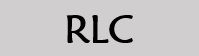This instruction holds significance when there exists a need to rotate the bits present in the accumulator. Basically, for an 8-bit value, each bit is rotated or shifted left by one position. Also, the rotation of last bit of the sequence i.e., D7, sets the CY flag.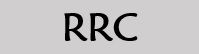This instruction rotates the bit to the right by one position. In this case, D0, sets the CY flag.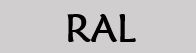This instruction rotates the bits to the left by one of the data present in the accumulator through the carry flag.

Here, D7 is shifted to carry the flag and the bit in the carry flag is shifted to D0.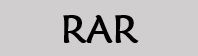This instruction is used to rotate the bits of the data present in the accumulator to the right through the carry flag.

In this case, D0 is shifted to carry the flag and carry bit is shifted to D7 position.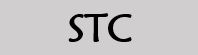This instruction sets the carry flag to 1, without affecting any other flags.This instruction is used to generate the complement of data at the accumulator. The complement function does not affect any of the flags.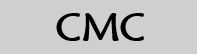This instruction complements the data present at the carry flag. Thus any other flag is not affected by this instruction.

### Branching Instruction

These instructions are used in order to switch or transfer the control of the microprocessor from one position to another. More specifically we can say, it changes the general sequential flow.

The branching instruction set is given below:This instruction is used to transfer the present program sequence to that memory location whose 16-bit address is specified in operand of the instruction.

Example: JMP 2020H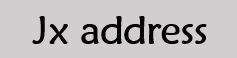This instruction comes under conditional branching instruction, in which the present program sequence is transferred to that particular location whose address is provided at the operand. But this transferring depends on the given PSX flag.

Example: JZ 1600H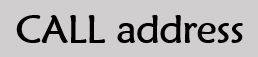This instruction moves the control of recent program sequence to the memory address given at the operand. But before transferring, the PC gets decremented.

Example: CALL 3400H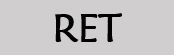This instruction causes unconditional return of the sub-routine to the actual program.This instruction is used to transfer the sequence from the main program to the interrupt service routine. Basically, the transfer is performed over one of the 8 bits which are specified in the operand.

### Control Instruction

As the name itself is suggesting that these instructions are used to control the operations of the microprocessor. The control instructions are as follows: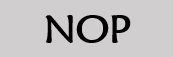It stands for no operation. Whenever the microprocessor fetches this instruction, then it is decoded but not executed.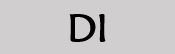It is used to disable the interrupts generated in the microprocessor. Resetting of interrupt enable flip-flop is performed. This leads to disabling of all the interrupts except TRAP.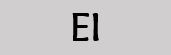This instruction is used to enable the interrupt. This time the interrupt enable pin is set which leads to enabling of the interrupts in the system.Whenever HLT is decoded by the microprocessor, then it halts any other program and continuously executes the current program which is under operation. To get out of the halt state either an interrupt or reset is required.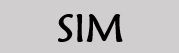SIM stands for set interrupt mask. This instruction is used to implement the programming of hardware interrupts 7.5, 6.5 and 5.5 and serial output.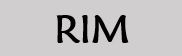RIM stands for read interrupt mask. This instruction is used to put the desired data at the accumulator according to the interrupt and serial input.

This is all about the instruction set of 8085 Microprocessor.